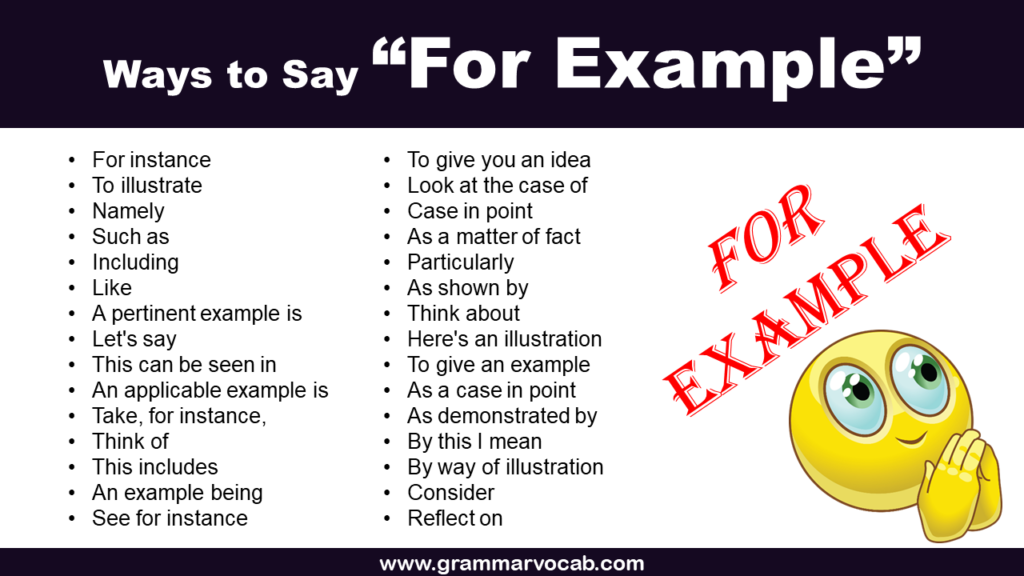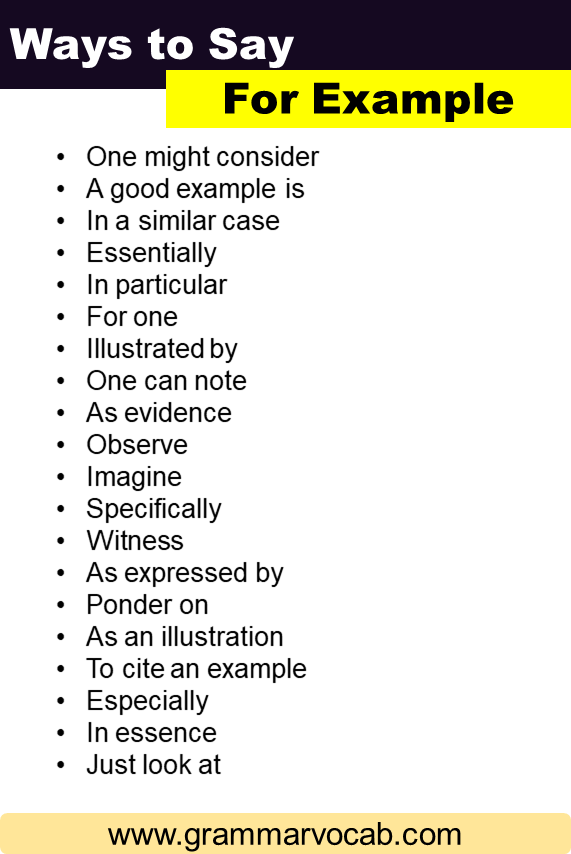# Other Ways to Say “For Example” in EnglishThe phrase “for example” is commonly used to introduce a specific instance or illustration of a point that one is trying to make. While it’s undoubtedly useful, sometimes variation in expression can make your writing or speech sound more engaging. Here’s a detailed breakdown of other ways to say for example that you can use in your writing and speech.

## Alternatives To For Example

• For instance
• To illustrate
• Namely
• Such as
• Including
• Like
• A pertinent example is
• Let’s say
• This can be seen in
• An applicable example is
• Take, for instance,
• Think of
• This includes
• An example being
• See for instance
• To give you an idea
• Look at the case of
• Case in point
• As a matter of fact
• Particularly
• As shown by
• Here’s an illustration
• To give an example
• As a case in point
• As demonstrated by
• By this I mean
• By way of illustration
• Consider
• Reflect on
• As seen in
• Demonstrated by
• A prime example of this is
• Exemplified by
• Effectively
• I mean
• In effect
• That is to say
• g. (abbreviation for “exempli gratia” in Latin, meaning “for example”)
• One example is
• One might consider
• A good example is
• In a similar case
• Essentially
• In particular
• For one
• Illustrated by
• One can note
• As evidence
• Observe
• Imagine
• Specifically
• Witness
• As expressed by
• Ponder on
• As an illustration
• To cite an example
• Especially
• In essence
• Just look at

Must Try:

Images